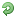##Write a print function

Hi
i did write a print function which the user put a number and then it will print out till that number

the output is correct ,, but is code correct ? or it could be better somehow ?

write a function named print_out that print all the whole numbers from 1 to n. test the function by placing it in a program that passes a number n to print_out , where this number is entered from the keyboard . the print_out function should have type void; it does not return a value. the function can be called with a simple statement:

print_out(n);

PHP Code:
``` # include <iostream> using namespace std; void print_out (int x); int main () {     int n;     cout << " enter a number : ";     cin >>n;     for ( int i=1; i<=n; i++)         print_out(i);     system ("pause");     return 0; } void print_out ( int x ) {     cout <<x;  }  ```